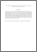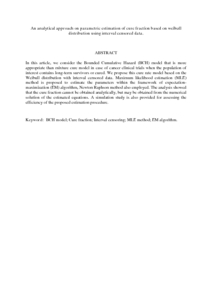# An analytical approach on parametric estimation of cure fraction based on weibull distribution using interval censored data.

## Citation

I. Aljawadi , Bader Ahmad and Abu Bakar, Mohd Rizam and Ibrahim, Noor Akma (2011) An analytical approach on parametric estimation of cure fraction based on weibull distribution using interval censored data. Australian Journal of Basic and Applied Sciences, 5 (3). pp. 546-554. ISSN 1991-8178

## Abstract

In this article, we consider the Bounded Cumulative Hazard (BCH) model that is more appropriate than mixture cure model in case of cancer clinical trials when the population of interest contains long-term survivors or cured. We propose this cure rate model based on the Weibull distribution with interval censored data. Maximum likelihood estimation (MLE) method is proposed to estimate the parameters within the framework of expectation-maximization (EM) algorithm, Newton Raphson method also employed. The analysis showed that the cure fraction cannot be obtained analytically, but may be obtained from the numerical solution of the estimated equations. A simulation study is also provided for assessing the efficiency of the proposed estimation procedure.Preview
PDF (Abstract)
An analytical approach on parametric estimation of cure fraction based on weibull distribution using interval censored data.pdfView Item